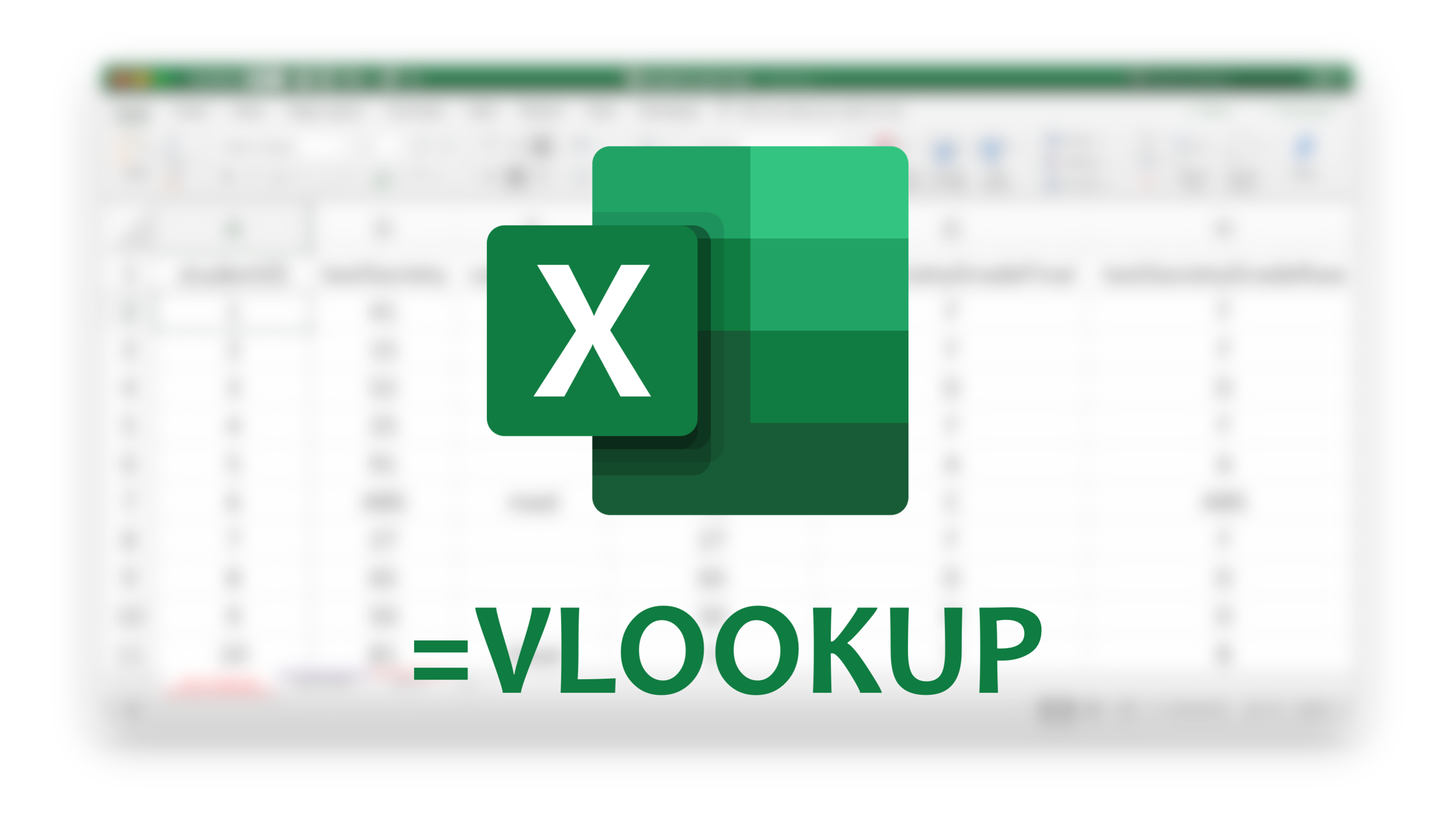# Excel to R #3 - APPROXIMATE VLOOKUP in Excel to FUZZY LEFT_JOIN in R

## using {fuzzyjoin}Despite all the memes around Microsoft Excel, Excel is still a powerful tool for quick and fast data transformation, data preprocessing (forget about the date thing 😉 ). This post is my attempt to tell an Excel person how they can replicate some of their most frequently used operation like VLOOKUP (Fuzzy) using R. We perform VLOOKUP’s approximate match first on Excel and replicate the same task on RStudio using stringdist_left_join() Fuzzy left join from the R package {fuzzyjoin}

In this video, We go through how to use the R package {fuzzyjoin} to replicate Excel’s popular VLOOKUP feature with approximate match

### Code

library(tidyverse)
library(fuzzyjoin)

sheet <- tibble::tibble(
Company = c("Apple Inc",
"Apple Incorp",
"Microsoft",
)

main <- tibble::tibble(
Company = c("Apple Inc",
"Microsoft",
Value = c(300,200,100,50)
)

left_join(sheet, main,
by = "Company")

stringdist_left_join(sheet,main,
by = "Company",
max_dist = 5)


If you still prefer to use Excel for Data Analysis - Check this out!

Related Posts: